### Commination Theory– AMS - Unit 1–Two Marks

Anna University

147402: Communication Theory

SEM / YEAR: IV/ II

QUESTION BANK

UNIT I

AMPLITUDE MODULATION SYSTEMS

PART A

1. State any two important spectral properties of periodic power signals.

Ans:

1. Linearity

2. Time shift.

3. Frequency Shift.

2. Define amplitude modulation. Give the expression for AM wave.

Ans:

It is the process by which the amplitude of the carrier wave is changed in Accordance with the instantaneous value of the message signal.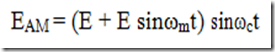3. A transmitter radiates 9 kW without modulation and 10.125 kW after modulation. Determine depth of modulation.

Ans: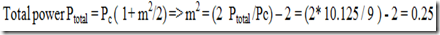Modulation index m = 0.5

4. A 500 W carrier is modulated to a depth of 60 percent. Calculate the Total power in modulated wave.

Ans:

5. Define VSB transmission. Mention its application.

Ans:

Definition:

One of the sideband is partially suppressed and portion of the other sideband is

transmitted. This portion compensates the suppression of the sideband. It is called vestigial sideband transmission.

Application:

VSB is mainly used in TV transmission

6. Give the applications of SSB-SC AM.

Ans:

1. Long distance point-to-point communications.

2. Mobile communications at frequencies below 30 MHz

3. It is also used in two way communications.

4. Multichannel communications in microwave.

7. Compare low level modulation and high level modulation.

Ans: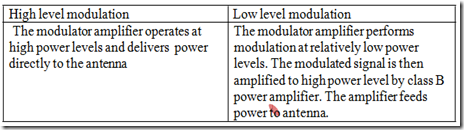8. An A M transmitter radiates 10 KW of power without modulation. With 0.65 depth of modulation what is the radiated power?

Ans:

9. Draw the frequency spectrum of VSB.

10. A carrier is amplitude modulated to a depth of 75 percent. Calculate the total Power in the modulated wave, if the carrier power is 40 watt.

Ans: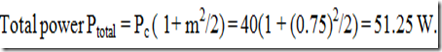11. Calculate the percentage of power saving when the carrier and one of the Sidebands are suppressed in an AM wave modulated to a depth of 100 percent.

Ans:

12. SSB is suitable for speech signals and not for video signals. Why?

Ans:

To generate SSB,the message spectrum must have an energy gap centered at the origin. This criteria is satisfied by speech signals, for which the energy gap is from -300 Hz to +300 Hz(600 Hz wide). Hence any one sideband can be easily isolated with the help of practical band pass filters. But in case of video signals, there is no such energy gap near origin. In other words, the video signal extends from DC origin. Hence lower and upper side bands are practically joined at origin. Hence with the help of practical band pass filters, it is not possible to isolate one sideband from other. Hence for video signals vestigial sideband transmission is suitable, rather than SSB.

13. A 2MHZ carrier having an amplitude of 5 V is modulated by a 4 kHZ audio signal having an amplitude of 2 V. Determine the modulation index.

Ans:

Modulation index m = Em / Ec = 2 / 5 = 0.4.

14. Define over modulation, under modulation and 100% modulation.

Under modulation. m<1

Critical modulation m=1

Over modulation m>1

15. Define modulation index of an AM signal.

Ans:

It is defined as the ratio of the maximum modulating voltage to the maximum carrier voltage. It is also called as ‘Depth of modulation’.

m = Vm/ Vc

16. Draw the spectrum of DSB.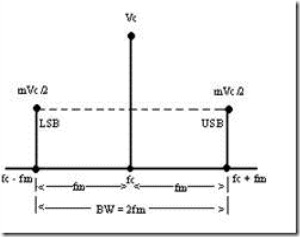17. Distinguish between low level and high level modulator.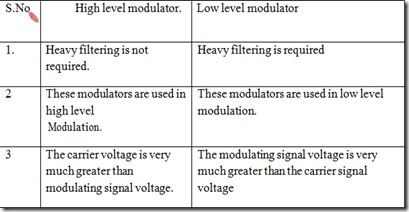18. Define FDM &frequency translation.

Ans:

Frequency translation:

The single sideband modulation basically performs frequency translation.

it is also called frequency changing , mixing or heterodyning. the single sideband modulation the message spectrum is shifted by an amount equal to the carrier frequency fc

FDM:

For voice transmission, the frequency range of 300 to 3400 Hz is used. when more number of such voice channels are to be transmitted, then each channel is given a fixed frequency slot and then transmitted. This is called Frequency Division Multiplexing.

19. What do you meant by image frequency?

Ans:

The local oscillator frequency (f0), input signal frequency (f) and IF (f) are related as, f0 – fs = f i.e., f0 = fs + fi

If some other frequency, f = fsi + 2fi appears at the input of mixer, then it produces fi at the output of the mixer. fsi- image frequency.

20. Why we need modulation?

Ans:

Needs for modulation:

1.Ease of transmission

2.Multiplexing

3.Reduced noise

4.Narrow bandwidth

5.Frequency assignment

6.Reduce the equipment's limitations.

21. When a signal m(t)=3 cos(2 103t) modulates a carrier c(t)=5 cos( 6t), find the modulation index and translate bandwidth if the modulation is AM.

Ans:

Modulation index m = Em / Ec = 3 / 5 = 0.6.

Bandwidth = 2 fm = 2 kHz

1.2.